Next: L-BFGS Minimiser Up: Optimisation of the Nudged Previous: Optimisation of the Nudged   Contents

## Quenched Velocity Verlet Minimiser

The QVV method is based on the velocity Verlet algorithm  (VV) as modified by Jónsson et al. , and was originally used for NEB optimisation. VV is a symplectic integrator that enjoys widespread popularity, primarily in molecular dynamics (MD) simulations where it is used for numerical integration of Newton's equations of motion. Its main advantage over the standard Verlet method is the minimisation of the round-off errors. At each time step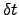the coordinates and the velocities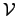are updated from the coupled first-order differential equations in the following manner :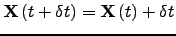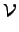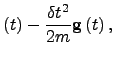(4.14)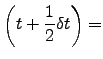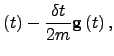(4.15)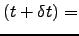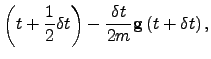(4.16)

where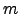is the atomic mass. The algorithm involves two stages, with a force evaluation in between. First the positions are updated according to Equation 2.14, and the velocities at midstep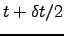are  then computed using Equation 2.15. After the evaluation of the gradient at time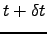the velocity is updated again [Equation 2.16] to complete the move. To obtain minimisation it is necessary to remove kinetic energy, and this can be done in several ways. If the kinetic energy is removed completely every step the algorithm is equivalent to a steepest-descent minimisation, which is rather inefficient. Instead, it was proposed by Jónsson et al.  to keep only the velocity component that is antiparallel to the gradient at the current step. If the force is consistently pointing in the same direction the system accelerates, which is equivalent to increasing the time step . However, a straightforward variable time step version of the above algorithm was reported to be unsuccessful .Next: L-BFGS Minimiser Up: Optimisation of the Nudged Previous: Optimisation of the Nudged   Contents
Semen A Trygubenko 2006-04-10